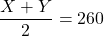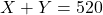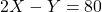Malcolm and Ravi raced each other. The average of their maximum speeds was 260 km/h If doubled, Malcolm’s maximum speed would be 80km/h end

Question

Malcolm and Ravi raced each other. The average of their maximum speeds was 260 km/h If doubled, Malcolm’s maximum speed would be 80km/h end text more than Ravi’s maximum speed. What were Malcolm’s and Ravi’s maximum speeds

in progress 0
5 months 2021-08-28T08:38:18+00:00 1 Answers 17 views 0

Given,

Average speed of Malcolm and Ravi = 260 km/h

Let speed of the Malcolm be X and speed of the Ravi Y.

From the given statement….(i)….(ii)

3 X = 600

X = 200 km/h

Putting value in equation (i)

Y = 520 – 200

Y = 320 Km/h

Speed of Malcolm = 200 Km/h

Speed of Ravi = 320 Km/h